## Introduction

A planar network is a graph that can be drawn on the two-dimensional plane such that no edges cross each other1. Planar graphs pervade many aspects of science: they are the subject of numerous studies in graph theory, in combinatorics2,3 and in quantum gravity4. Planar graphs are also central in biology where they can be used to describe veination patterns of leaves or insect wings. In particular, the vasculature of leaves5,6 displays an interesting architecture with many loops at different scales, while in insects, the vascular network brings strength and flexibility to their wings. In city science, planar networks are extensively used to represent, to a good approximation, various infrastructure networks7. In particular, transportation networks8,9 and more recently streets patterns10,11,12 are the subject of many studies13,14,15,16,17,18,19,20,21,22,23,24,25,26,27,28,29 that are trying to characterize both topological (degree distribution, clustering, etc.) and geometrical (angles, segment length, face area distribution, etc.) aspects of these networks.

Despite a large number of studies on planar networks, there is still a lack of global, high-level metrics allowing to characterize their structure and geometrical patterns. Such a characterization is however difficult to achieve and in this article, we will discuss an important aspect of planar graphs which is intimately connected to their geometrical organization. In this respect, we will define new metrics and test them on various datasets, both artificial (roads, highways, railways and supply networks) and natural (veination patterns of leaves and wings, slime mould) enabling us to obtain new information about the structure of these networks.

We will now introduce the main metrics used in this article. Generally speaking, we can define different types of paths for a given pair of nodes (i, j). A usual quantity is the shortest euclidean path of length ℓ(i, j) which minimizes the distance travelled to go from i to j. We can however ask for another path which minimizes the number of turns - the simplest path, of length ℓ*(i, j) (if there are more than one such path we choose the shortest one). Fig. 1a displays an example of the shortest and simplest path for a given pair of nodes on the Oxford (UK) street network.

To identify the simplest path, we first convert the graph from the primal to the dual representation, where each node corresponds to a straight line in the primal graph. These straight lines are determined by a continuity negotiation-like algorithm, as described in Material and Methods. Edges in dual space, in turn, represent the intersection of straight lines in the primal graph (see Fig. 1b).

We define the number of turns τ of a given path as the number of switches from one straight line to another when walking along this path. This quantity is intimately related to the amount of information required to move along the path14. We have computed the probability distribution P(τ) for all shortest and simplest paths of several networks (see SI) and the results show that this distribution is usually centered around a smaller values for the simplest paths than for the shortest paths, as expected. More generally, we show in the Supplementary Information that the average number of turns 〈τ〉 versus the number of nodes N indeed displays a small-world type behavior characterized by a slow logarithmic increase with N, consistently with previous analysis of the dual network14,16. This feature is thus not very useful to distinguish different networks and shows that the distribution of the number of turns is a very partial information and tells very little about the spatial structure of the simplest paths. For navigation purposes (neglecting all congestion effects) and in order to understand the structure of the network, it is useful to compare the lengths of the shortest and the simplest paths with the ratio ℓ*(i, j)/ℓ(i, j) ≥ 1. It is then natural to introduce the simplicity index S as the average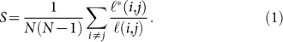The simplicity index is larger than one and exactly equal to one for a regular square lattice and any tree-like network for example. Large values of S indicate that the simplest paths are on average much longer than the shortest ones and that the network is not easily navigable. We note here that we do not take into account congestion effects which can influence the path choice (see for example30). This new metric is a first indication about the spatial structure of simplest paths but mixes various scales and in order to obtain a more detailed information, we define the simplicity profile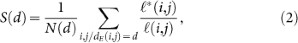where dE (i, j) is the euclidean distance between i and j and where N(d) is the number of pairs of nodes at euclidean distance d. This quantity S(d) is larger than one and its variation with d informs us about the large scale structure of these graphs. We can draw a generic shape of this profile: for small d, we are at the scale of nearest neighbors and there is a large probability that the simplest and shortest paths have the same length, yielding S(d → 0) ~ 1 and increasing for small d. For very large d, it is almost always beneficial to take long straight lines when they exist, thus reducing the difference between the simplest and the shortest paths. As a result we expect S(d) to decrease when ddmax (note that a similar behavior is observed for another quantity, the route-length efficiency, introduced in31). The simplicity profile will then display in general at least one maximum at an intermediate scale d* for which the length differences between the shortest and the simplest path is maximum. The length d* thus represents the typical size of domains not crossed by long straight lines. At this intermediate scale, the detour needed to find long straight lines for the simplest paths is very large.

We finally note here that these indices are actually not limited to planar networks but to all networks for which the notion of straight lines has a sense and can be computed. This would be the case for example for spatial networks which are not perfectly planar7.

We introduce a null model in order to provide a simple benchmark to further analyze the results obtained by these new metrics (the expression ‘null model’ should be understood here in the sense of the benchmark and not in the usual statistical definition). The goal in this study is to compare empirical results with a very simple model based on a minimal number of assumptions, but we note that it would be also interesting to compare various models generating planar networks. We start with N points randomly distributed in the plane and construct the Voronoi graph (see the Supplementary Information for further details). We then add a tunable number of straight lines of length ℓ distributed according to P(ℓ) ~ ℓ−α. Examples of networks generated by this model as well as many results are shown in the SI.

We first study static networks (see Fig. 2) such as the streets of cities (Bologna, Italy; Oxford, UK; Nantes, France), the national highway network of Australia, the national UK railway system and the water supply network of central Nantes (France). In the case of biological networks, we study the veination patterns of leaves (Ilex aquifolium and Hymenanthera chatamica) and of a dragongly wing. Details on these datasets can be found in the SI.

We also consider three datasets describing the time evolution of networks at different scales (see Fig. 3): at a small scale and in the biological realm we study the evolution of a slime mould network. At the city scale we present results on the road network of Paris (France) from 1789 until now. Paris was largely transformed by a central authority (the prefect Haussmann under Napoleon III) in the middle of the 19th century and the dataset studied here displays the network before and after these important transformations, offering the possibility to study quantitatively the effect of top-down planning28. At the multi-town level, we study the road network of the Groane area (Italy) (see the SI for details on these datasets). These networks allow us to explore different systems at very different scales from 10−3 (Slime mould) to 106 (Australian highways) meters.

We compute the simplicity index S for the various datasets and for the null model as well. The results are shown in Fig. 4 as a function of the density of straight lines ρ and the Gini coefficient G for the length of straight lines (see Material and Methods for details). The density ρ of straight lines is defined as the ratio of total length of straight lines (see Fig. SI2), over the total length of the network and G is an indicator of the diversity of the length of straight lines.

The first observation from Fig. 4 is that the simplicity index encodes information which is neither contained in the density ρ nor in the Gini coefficient G and reveals how the straight lines are distributed in space and participate in the flows on the network.

In Fig. 4a, we observe that the density of straight lines is always larger for urban systems. More precisely, in the biological systems the density lies in the range ρ [0.55, 0.7], while we observe ρ > 0.7 for artificial systems. Except for the Physarum, which appears to be close to a regular lattice with a small simplicity and small Gini coefficient, the simplicity index for the wing and the leaves is larger than the values obtained for the null model. These results indicate that the organization of straight lines in biological systems is very different from artificial systems, that have very similar values of ρ, G and S. In particular, we observe a hierarchy of straight lines in biological systems (see Fig. 2): a main artery (the midrib for leaves) connects to veins which in turn are connect to smaller veins and so on. In the case of dragonfly wing, the main straight line is given by the external border of the network. The existence of these main straight lines in biological systems will impact the structure of simplest paths and impose some large detour, resulting in a larger value of the simplicity index.

For urban systems, the simplicity is very close to the null model (of order 1.3 in this density range), suggesting that in dense urban systems, long straight lines are added at random (An exception concerns, the pre-Haussmannian Paris (1789–1836) for which we observe a simplicity smaller than for the null model, the reason being probably that the networks at these times were very sparse). As a result, navigation on urban systems requires relatively less information with no additional cost: the simplest path is not too different from the shortest path.

Finally, we note an interesting effect in the null model in Fig. 4a which is the existence of a maximum of the simplicity at densities of order ρ ~ 0.55. In this density regime, using straight lines implies having to make large detours. However, when the density exceeds 0.6, there are enough straight lines to enable a simplest path which differs not too much from the shortest one.

We now discuss the simplicity profile shown in Fig. 2. We observe that basically for most of these systems, the simplicity profile displays the generic shape with a maximum at an intermediate scale. In urban cases, such as Bologna and central Nantes, we have a typical monocentric system with a dense center and a few important radial straight lines, leading to a simple profile S(d). In the case of Oxford and the Australian highway network, the polycentric organization leads to multiple peaks in the simplicity profile (Fig. 2). Interestingly, we observe that the profiles for australian highways and railways in the UK are very different, despite their similar scale, density ρ and Gini coefficient G. In particular, the UK railway displays small values of the simplicity (less than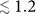) while for the Australian highway network there are many pairs of nodes for which the simplest path is much longer than the shortest one. We also observe that the profile for both street and water systems of Nantes have a very similar shape, pointing to the fact that these networks are strongly correlated. In addition, the position and the height of the peak (≈ 1.4) observed for the Nantes water system suggests that this distribution system has similar features compared to biological systems such as vein networks in leaves (see below) whose function is also distribution.

Compared to urban systems, the simplicity profile of biological networks have a single well-defined and much more pronounced peak. We observe values of order Smax ≈ 1.5 and 2.5 for d*/dmax ≈ 0.2, meaning that for this range of distance, the detour made by the simplest path is very large. This peak is related to the existence of domains of typical size d* not crossed by large veins. We see here a clear effect of the existence of the spatial organization of long straight lines in these systems, probably optimized for the distribution (of water for leaves). The decay for large d is also much faster in the biological case compared to urban systems: this shows that in biological systems there are long straight lines allowing to connect far away nodes. This is particularly evident on the leaves shown in Fig. 2 where we can see the first levels (primary and secondary) veins, the rest forming a network. For streets, the organization is much less rigid and the hierarchy less strict: we have a more uniform spatial distribution of straight lines, leading to a smoother decrease of S(d).

Going beyond static networks, we apply our new metrics to study the structural changes of time evolving networks.

The first example of a time evolving network is the road network of the Groane region which is a 125 km2 area located north of Milan26. We have 7 snapshots of this network for different times from 1833 to 2007 (see the Supplementary Information for details). This region evolved without central planning and is thus a good example of an ‘organic’ evolution of urban systems. The simplicity profile shown in Fig. 3(a) allows us to distinguish two different periods. The first period from 1833 to 1955 displays a relatively small simplicity at all scales, while a distinct second regime appears from 1980 until now. In this latter regime, the simplicity profile is substantially larger for all scales. This is an effect of the massive urban densification, leading to a polycentric structure where the readability and the ease to navigate are drastically lowered.

At a smaller scale, we study the evolution of central Paris between 1789 and 1999. This dataset provides an interesting case study, as Paris experienced larges changes due to Haussmann in the middle of the 19th century (see28 for details and more references about this network). This is an opportunity to observe quantitatively the effect of top-down planning: until 1836, we are in the pre-Haussmann Paris, while from 1888 until now we are in the post-Haussmann period. The effect of Haussmann's central planning is clearly visible on the network shown in Fig. 3(b). From 1789 to 1836, we have a relatively large simplicity at all scales and we observe a decrease in that period at small scales (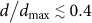) which corresponds well to the fact that many religious and aristocratic domains and properties were sold and divided in order to create new houses and new roads, improving congestion inside Paris. The 1826–1836 transition displays a decrease of the simplicity for distance larger than roughly 5 kms (corresponding to d/dmax ≈ 0.6) indicating that long distance routes were simplified. It is interesting to note that during this period the eastern part of Paris experienced large transformations with the construction of the channel St. Martin. Finally in the period 1836 to 1888, when Paris experienced Haussmann's transformation, the simplicity profile is strongly affected: compared to 1836, the simplicity is improved in the range d/dmax [0.3, 0.8], which can be attributed to the construction of large avenues connecting important nodes of the city. In addition, we observe the surprising effect that at large scales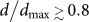, the simplicity is degraded by Haussmann's work: this however could be an artifact of the method and the fact that we considered a portion of Paris only and neglected the effect of surroundings.

We also note that differences between Groane and Paris might be explained in terms of a sparse, polycentric urban settlement (Groane) versus a dense one (Paris). In particular, in the ‘urban’ phase for Groane (after 1955), the simplicity profile becomes similar to the one of a dense urban area such as Paris.

Finally, we show the results in Fig. 3(c) for the Physarum Policephalum, a biological system evolving at the centimeter scale. Physarum is a unicellular multinucleated amoeboid that during its vegetative state takes a complex shape. Its plasmodium viscous body whose goal is to find and connect to food sources, crystallizes in a planar network-like structure of micro-tubes33. In simple terms, Physarum's foraging strategy can be summarized in two phases: i) the exploration phase in which it grows and reacts to the environment and ii) the crystallization phase in which it connects to food sources with micro-tubes. We inoculated active plasmodium over a single food source and observe the micro-tube network at six phases of its growth (see SI for details). Under these conditions, we observe that the network is statistically isotropic around the food source as shown in Fig. 3(c) and develops essentially radially. We first observe that the simplicity profile for the Physarum is relatively low (less than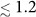), suggesting that simplicity could be an important factor in the evolution of this organism. A closer observation shows that during its evolution, the Physarum adds new links to the previous network and also modifies the network on a larger scale, as revealed by the changes of the simplicity profile. The evolution of the profile is similar to the one obtained for the null model when the density is increased (see SI), suggesting that the statistics of straight lines in this case could be described as essentially resulting from the random addition of straight lines of random lengths (with α = 2).

We have shown that the new metrics introduced here encode in a useful way both topological and geometrical information about the global structure of planar graphs. In particular, our results highlight the structural differences between biological and artificial networks. In the former, we have a clear spatial organization of straight lines, with a clear hierarchy of lines (midrib, veins, etc), leading to simplest paths that require a very small number of turns but at the cost of large detours. In contrast, there is no such strong spatial organization in urban systems, where the simplicity is usually smaller and comparable to a null model with straight lines of random length and location. These differences between biological and urban systems might be related to the different functions of these networks: biological networks are mainly distribution networks serving the purpose of providing important fluids and materials. In contrast, the role of road networks is not only to distribute goods but to enable individuals to move from one point of the city to another. In addition, while biological networks are usually the result of a single process, urban systems are the product of a more complex evolution corresponding to different needs and technologies.

These new metrics also allow us to track important structural changes of these networks. The simplicity profile thus appears as a useful tool which could provide a quantitative classification of planar graphs and could help in constructing a typology of leaves or street patterns for example.

## Methods

* Measuring the simplest path All the simplest paths of a given network were calculated in the dual space by converting the networks from the primal to the dual representation, where straight lines are mapped into nodes and the intersection between straight lines were mapped into edges. Straight lines are found by using a version of the ICN (Intersection Continuity Negotiation) algorithm16. More specifically, given an edge (i, j), we search among the adjacent edges attached to j, (j, k), that one that is most aligned to (i, j). If the angle θi,j,k between (i, j) and (j, k) is smaller or equal to θc = 30°, we assume that these two edges belong to the same straight line. This procedure continues until no more edges are assigned to the same straight line. Then, the procedure is repeated in opposite direction starting from the adjacent edges attached to node i. Once assigned to a straight line, an edge is removed from the network. As it is, this algorithm produces different networks depending on the choice for the initial edge. To overcome this ambiguity, our algorithm always starts with the edge that give us the longest straight line for a given network. After this straight line is fully detected and its edges deleted, we choose the next edge that will give us the second longest straight line and so on. The algorithm ends when there are no more edges left in the network.

Once all straight lines have been identified, the dual representation is built by looking at the intersection between straight lines. Each straight line is mapped onto a node in the dual space and two nodes are connected together if their respective straight lines intersect each other at least once. To illustrate this process, we show in Fig 1(b,1) an example of planar network in the primal representation where the edges are colored according the id of the straight line they belong to. In Fig. 1(b,4) we show the dual representation of the same network. It is important to note that the longest straight lines, in this example represented by orange, red, green and magenta give rise to hubs in the dual space.

In order to calculate the simplest path between nodes 1 and 2 from Fig. 1(b,1), we search for the shortest path between their respective straight lines in the dual space, cyan and blue in this case. As it can be seen, there are two paths with length 4, A and B. Each of them define a subgraph in the primal representation, here represented by the set of magenta lines in Fig. 1(b,2) and red lines in Fig. 1(b,3) for paths A and B, respectively. Then we evaluate the shortest path distance between nodes 1 and 2 over these subgraphs and we adopted the shortest one as the simplest path - green dashed in Fig. 1(b,3). The black path in Figs. 1(c,d) represent the shortest path between nodes 1 and 2.

* Gini coefficient The Gini coefficient quantifies the inequalities of the lengths of straight lines and is defined as in32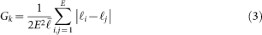whereis the average length of straight lines and E is the number of straight lines. The Gini coefficient lies in the range [0, 1] and G = 0 when all lengths are equal. On the other hand, if all lengths but one are very small, the Gini coefficient will be close to 1.